## Random Number Generation Part II – Some Data Analysis

Following on from my last post, I’ve been trying to read the voltage values that are generated from the random number generator I put together. I was initially using an arduino unit so I could control the voltage output down to below 1 volt. Ultimately I want to read the values with an ESP8266 unit running micropython so I can upload the values to an internet based data logger and the ESP8266 is only 1 volt tolerant on its analog-to-digital pin. So, the voltage has to be controlled and I didn’t mind blowing up one of my arduinos (which are 5 volt tolerant). The voltage divider is now composed of a 10k resistor from positive rail to analog out and a 1k resistor from analog out to ground.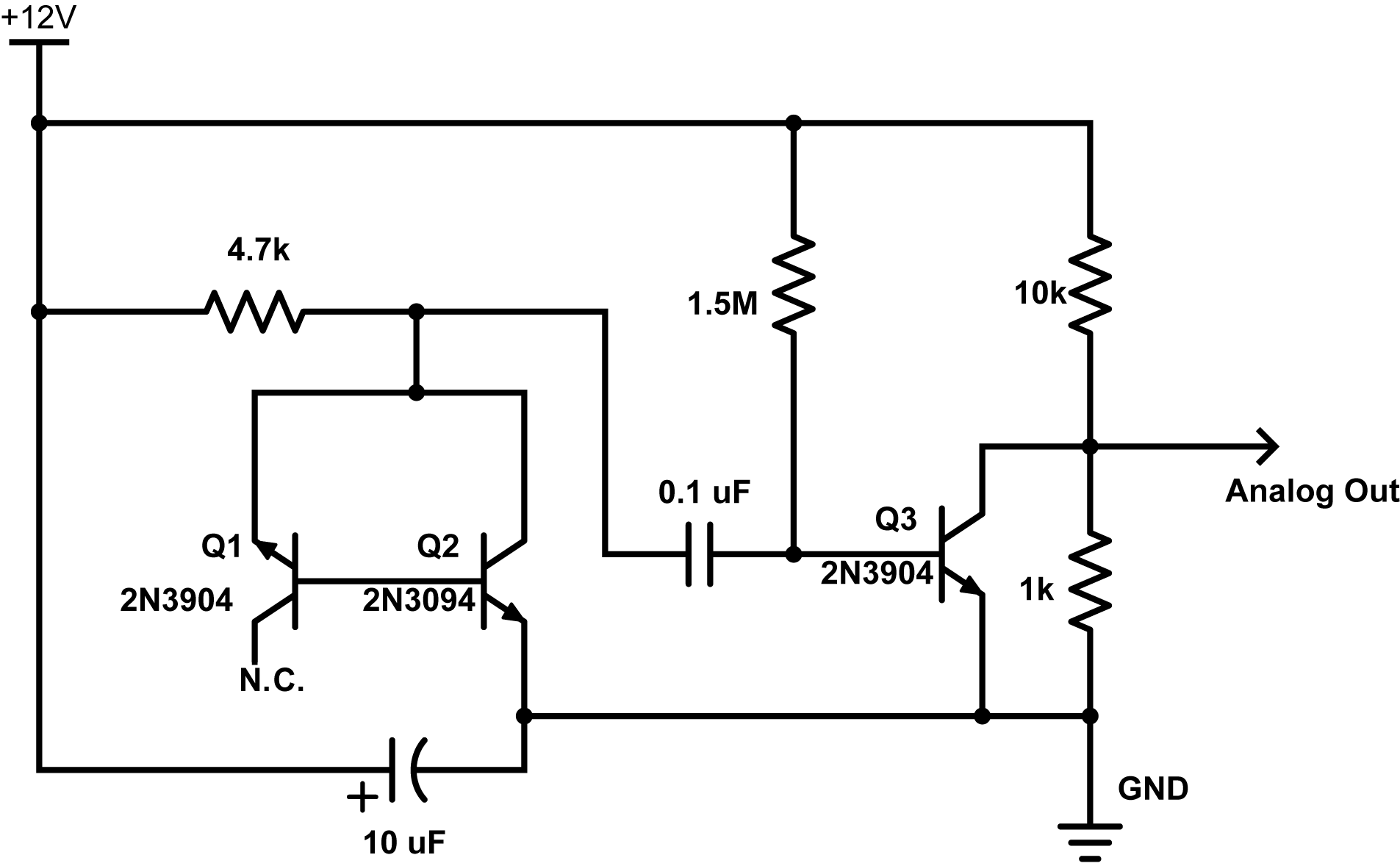The ardunio was giving me readings up to about 180 unit out of 1024 which gives about 180/1024 * 5V = 0.8789 volts… nice and safe.

Reading these voltages with the ESP8266 gave some interesting results. The code to do this was very simple:

```from machine import ADC

import time

for i in range(1000000):
time.sleep_ms(10)```

This reads a voltage every 10 milliseconds from the analog pin and reports one million of them. It took about 2.8 hours and the output was redirected to a file of type csv with this command on my mac:

`ampy --port /dev/tty.SLAB_USBtoUART run readanalog.py > avalanche_noise.csv`

‘ampy’ is a nice program from Adafruit, which you can learn more about here.

I then analyzed this data in python using the jupyter web interface. A histogram plot of the values looks like this: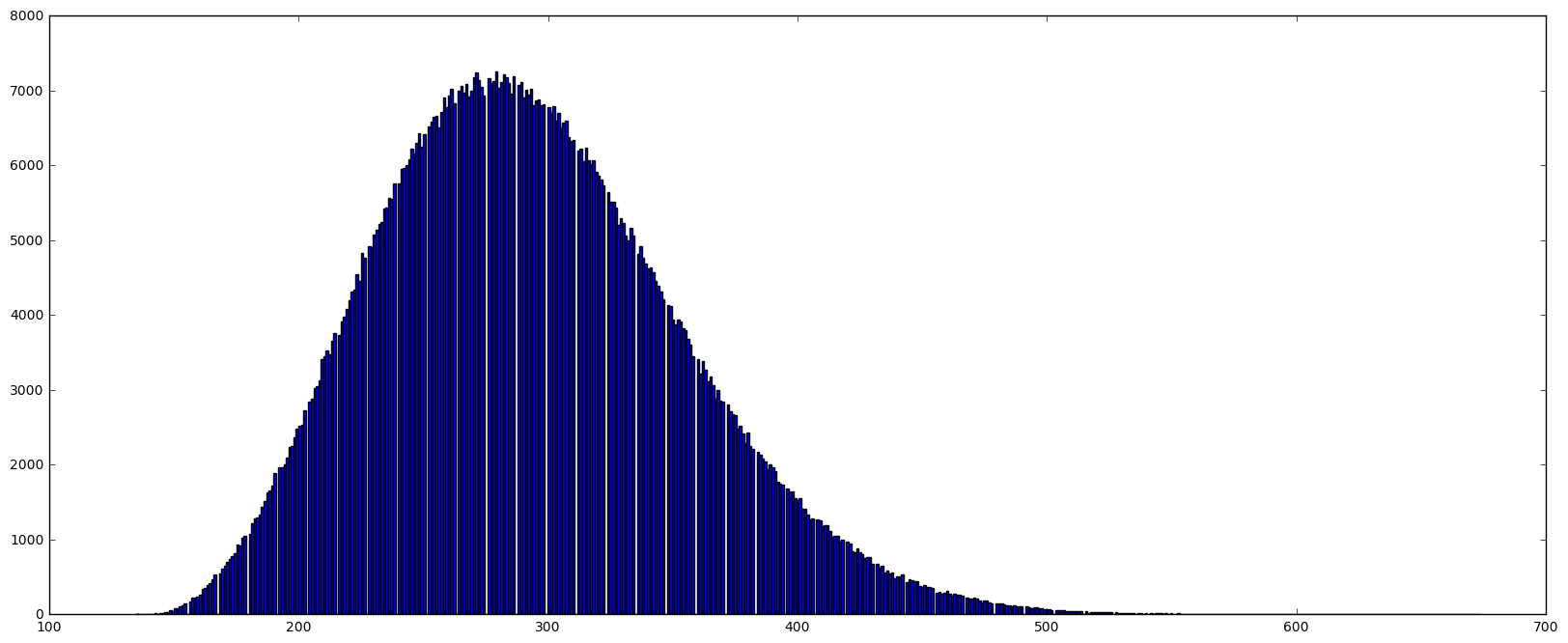The values range from 135 to 674 with a mean of ~292.6. This distribution is obviously skewed too so it’s not gaussian distributed – maybe Poisson?  But the most striking thing is the lines of empty space (no blue) – is this a plotting problem or are certain values skipped during the reads? Well lets zoom in around the top of the distribution.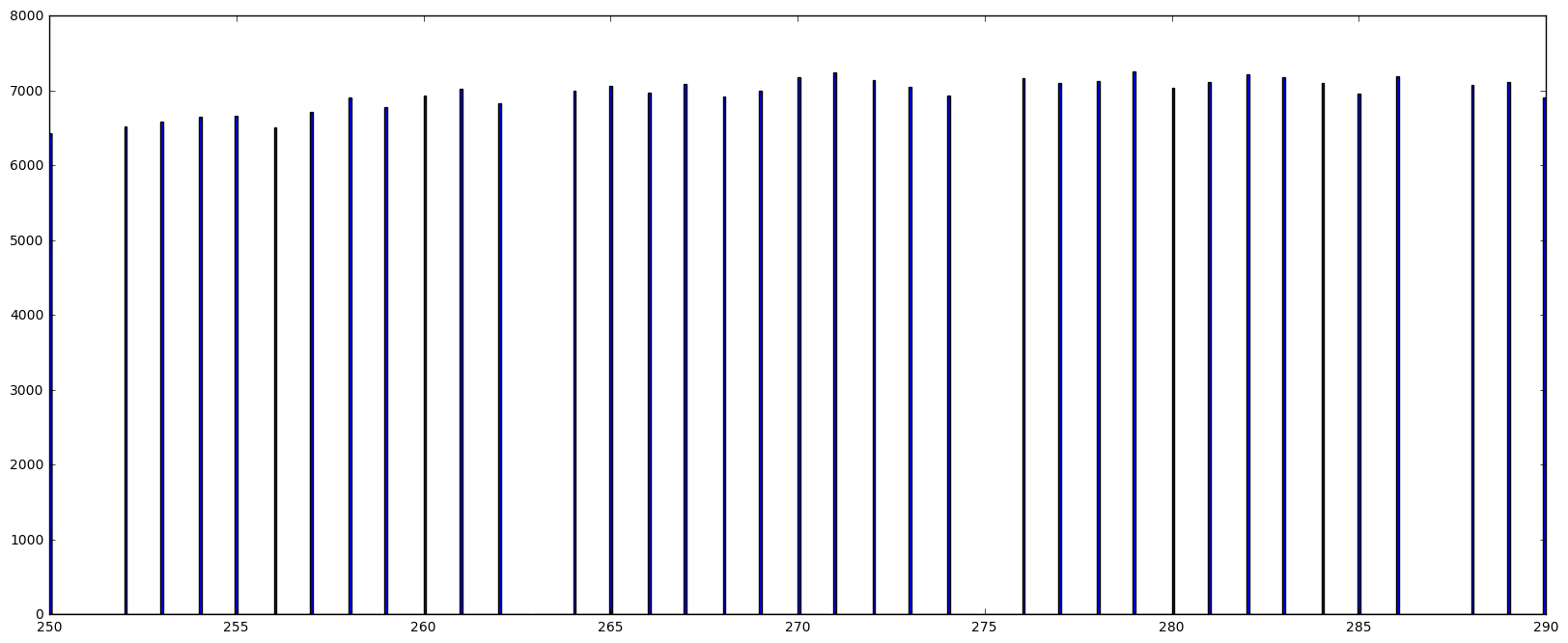So its true, some values are just never recorded. Its hard to believe the voltages from the circuit would do this. Also, its clear from the numbers that every 12th value is skipped. I think this must be an error in the way micropython reads the register so I’ll probably post this somewhere in the micropython forums and see if this is true or if I’m just doing something wrong.

Next I tried to fit this distribution to a gaussian curve. It failed. Here is the python code:

```import math
import numpy as np
import matplotlib.pyplot as plt
import scipy as scipy
import scipy.stats as stats
from scipy.optimize import curve_fit
from scipy.misc import factorial
from scipy.stats import norm
%matplotlib inline          # needed for plotting in jupyter

data_p = data # not really needed

fig = plt.figure(figsize=(20, 8))
entries, bin_edges, patches = plt.hist(data_p, bins=(int(np.amax(data_p)-np.amin(data_p))), range=[np.amin(data_p),np.amax(data_p)], normed=True)
bin_middles = 0.5*(bin_edges[1:] + bin_edges[:-1])

# poisson function, parameter lamb is the fit parameter
def gauss(x, *p):
A, mu, sigma = p
return A*np.exp(-(x-mu)**2/(2.*sigma**2))

# fit with curve_fit
p0 = [1., 260., 30.] # initial guesses for height, mean and SD

parameters, cov_matrix = curve_fit(gauss, bin_middles, entries, p0 = p0)
print parameters # print results
print np.sqrt(np.diag(cov_matrix)) # print errors of fit

# plot poisson-deviation with fitted parameter
x_plot = np.linspace(0, np.amax(data_p), 1000)
plt.plot(x_plot, gauss(x_plot, *parameters), 'r-', lw=4)
plt.show()```

Output: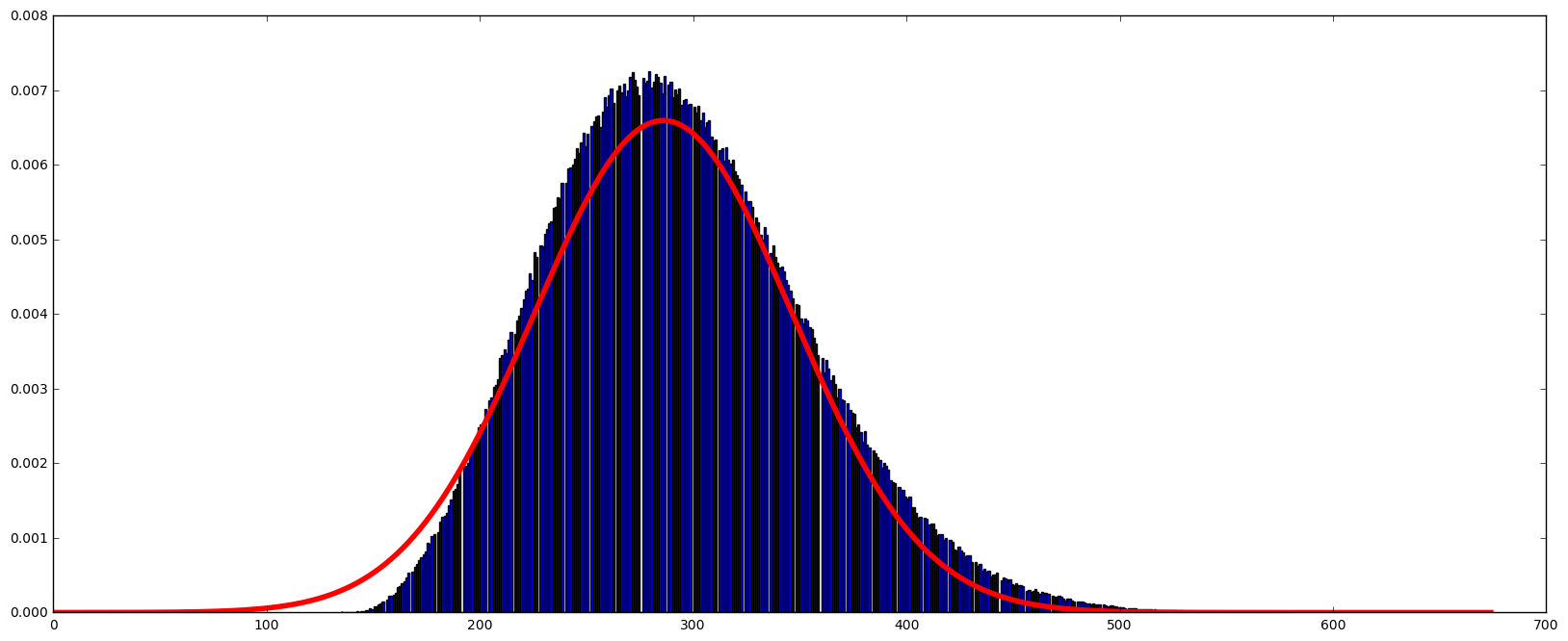This doesn’t fit very well. But it does reveal the skew present in the data. It does in some ways look poisson distributed (you can read more about this distribution here) and electrical noise is typically poisson distributed because of the discrete nature of electrical charge (read about this and shot noise here).

Mathematical aside:

One way I like to think of the Poisson distribution and Poisson processes is as follows. They arise from discrete events that must always have a positive value – this is not true for Gaussian distributions. So, in our circuit, current (electrons) flow or do not flow. When they flow, a discrete (integer) number of them flow. There is a lower limit on the number that can jump the gap. That number is zero. The gaussian distribution is the limit of a binomial distribution as the number of events goes to infinity. The binomial distribution is based on the idea that an event either happens or does not. (N.B. this is different from the Poisson case because in that case, events either happen 1 or more times or do not happen). If we accumulate binomial events, there is no limit to how many ‘yes’ or ‘no’ events can happen so some of the distribution must extend as far as the number of events recorded – for gaussian this limit goes to infinity and negative infinity. The Poisson distribution doesn’t act this way. If we try and model the Poisson distribution as an infinite binomial distribution we quickly realize that while we can get an infinite number of ‘zero value’ events as well (with low probability) there is more than one other alternative. So the distribution must take into account these many possible values which stretches the distribution in the positive direction while there is a still a hard limit at zero. We can shift the Poisson distribution so ‘N’ zero-value trials will be plotted at ‘-N’ (like we would for a binomial distribution) but on the positive size, the curve would extend past ‘+N’ because some of those trials can have a value of more than 1.

Enough qualitative description of distributions…

Using a poisson function instead like this:

```def poisson(k, lamb):
return (lamb**k/factorial(k)) * np.exp(-lamb)```

and we get this: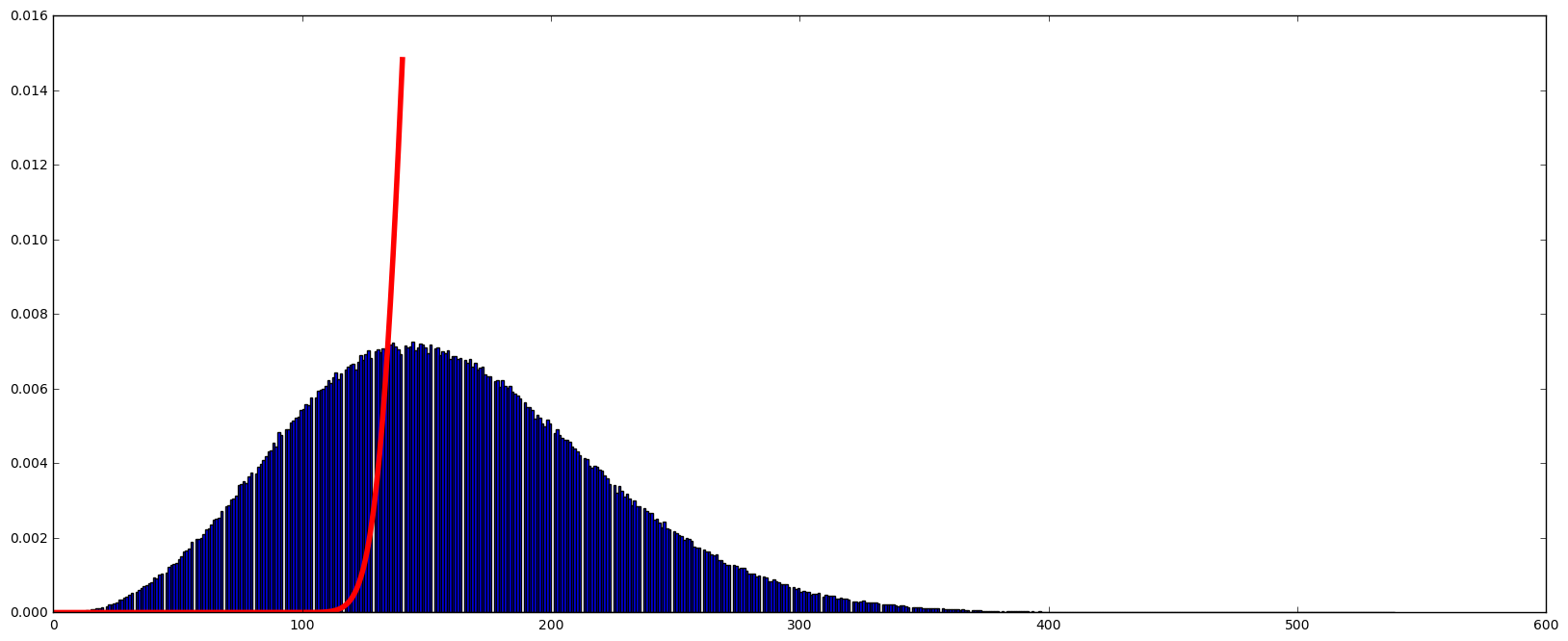It doesn’t converge at all.

Yikes, whats going on?

Well my thought was that the numbers I’m reading don’t match the number of electrons actually flowing. This signal is amplified by the transistors and then quantized, not in nature, but by the ADC converter in the ESP8266. My hope is that this signal is proportional to the number of electrons that flowed during signal acquisition. But this signal is not the same as the number of electrons which will be behaving as a Poisson process. But the recorded numbers should be proportional to the number of electrons. So if we divide these values by some constant, can we get the fit to work, and at what optimal division factor.

Long story short, if I divide the 1000000 million points by 23.9 I get an optimal fit in terms of the error reported for the fitted parameter. That parameter, which is the mean value, is ~6.558336. Does this mean, that on average 6.5 electrons pass through the transistor while the ESP8266 is taking an ADC measurement? I think it might be! Here is the fit: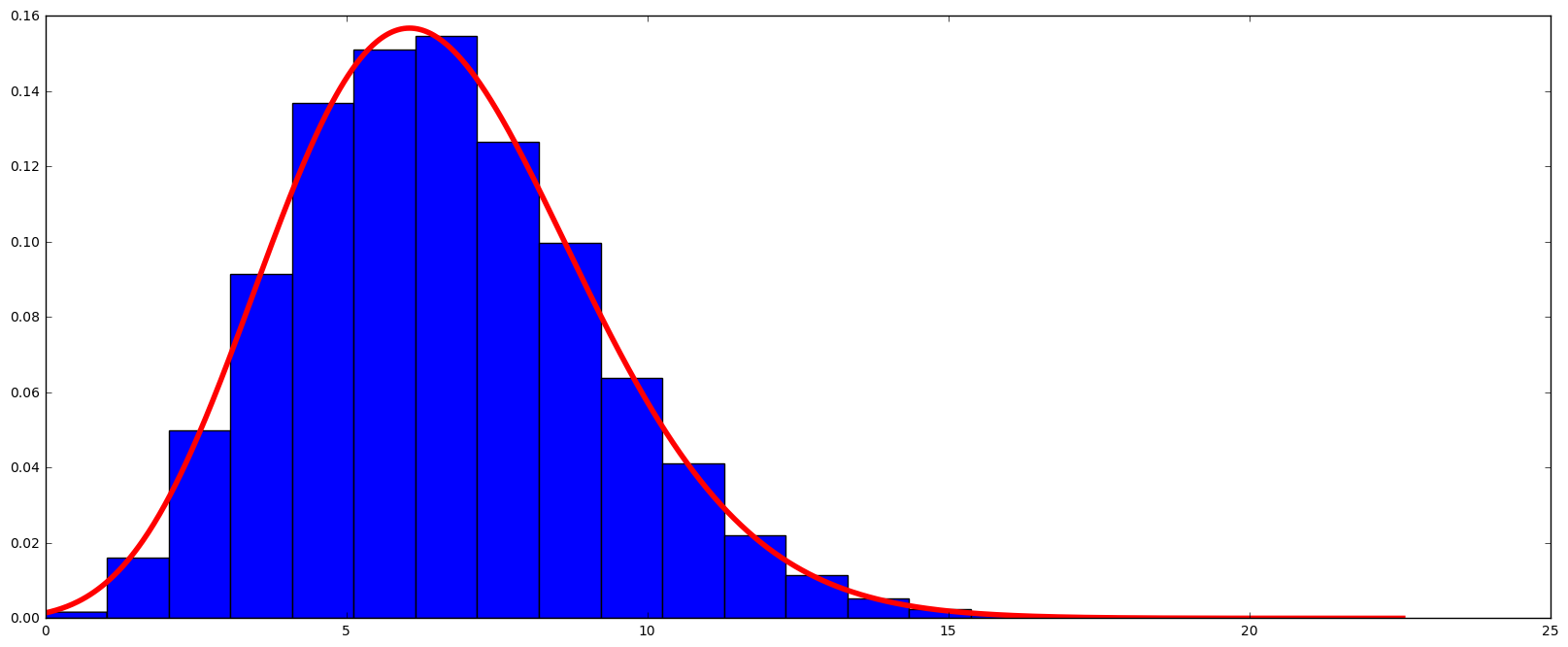If I take these same numbers and try and fit a Gaussian curve, it doesn’t do as well.Conclusions? The electrons that flow across the junction in reverse bias are behaving as a Poisson process as expected. The distribution is not flat. I’ve seen some discussion on the net where people seem to assume this would be the case. It does seem to be random! One of the next steps is to convert these numbers to a flat distribution or at least make it generate a binary sequence. It seems to be that XORing  or Von Neumann filtering will not do a good job of removing the biasing that the Poisson distribution will introduce.

## Random Number Generation Part I – The Hardware

Intro:

The notion of randomness has consistently intrigued me, so I have always wanted to build a random number generator and play with it. Just how easy is it to generate truly random numbers as opposed to pseudo-random numbers? First of all, pseudo-random numbers are based on an algorithm so computationally they are easy to generate but also easy to copy or determine the nature of the sequence. They also ‘repeat’ their pattern eventually, even if the repeat cycle might be very large. No, no, no. I want to generate random numbers from an unpredictable natural source such as radioactive decay, cosmic rays, radio noise or ‘avalanche noise’ (hint: not the noise of snow falling down a mountain).

The Source:

In short, avalanche noise is the noisy current flow when a diode is reverse biased (voltage applied the wrong way), once that voltage is high enough to make electrons jump over the semiconductor gap the wrong way.  The nifty thing is transistors have these diode junctions and so current can flow, for example, from the base to the emitter in an NPN transistor once it is reverse biased with high enough voltage. So what many circuits do to generate random noise is set up a transistor in this way, and then amplifying the current that flows, which for ‘quantum energy gap’ reasons is noisy.

An Example Circuit:

Browsing the net I came across Rob Seward’s attempts at doing this and set up his circuit since I had all the components on hand. The circuit is below: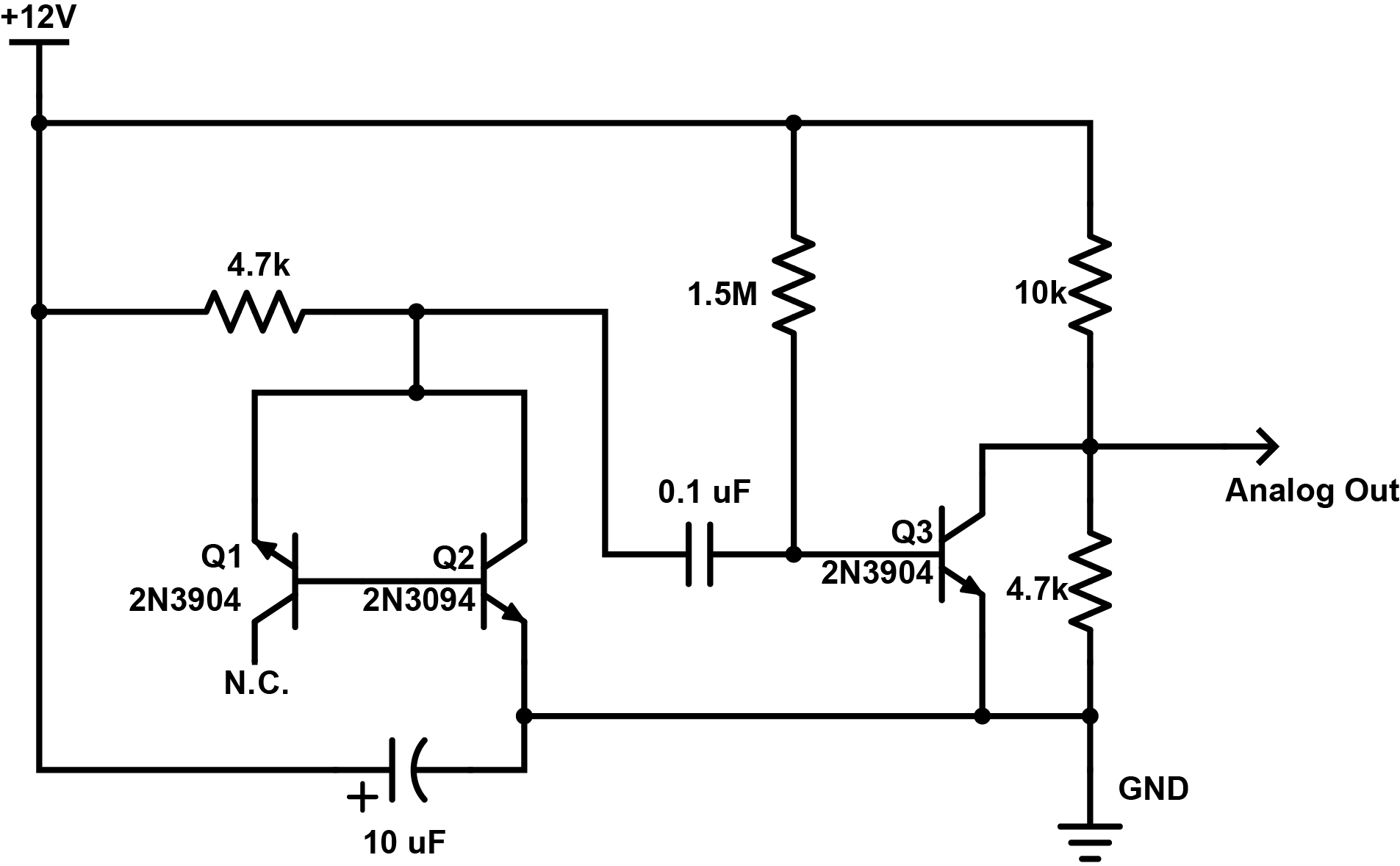This is my understanding of this circuit: Here, Q1 is reverse biased with 12 V of EMF via the 4.7k resistor. Q2 is forward biased and so it should conduct with a small voltage drop (~0.9 V) across its base to emitter junction so the reverse bias in Q1 is actually ~11.1 volts through the 4.7k resistor. This should be enough to jump the gap as outlined in this great summary of this phenomenon by Giorgio Vazzana. If current randomly jumps the gap in Q1, this current will flow into the base of Q2 where it will be amplified by a common emitter setup and passed into the 0.1 uF capacitor. These spikes in current will pass through the capacitor and into the base of Q3, which is highly biased by the 1.5M resistor. I think whats going on here is this transistor is set up to be a switch – this high bias turns the collector to emitter current essentially off, so any current that flows into its base will turn it on. Thus a voltage appears at its collector (its also in common emitter mode). This voltage is divided by the two resistors of 10k and 4.7k and presented as an analog out signal.

I put this together and applied the analog out and a ground to some earbuds I had lying around. Faint noise!

I quickly wired up an arduino to take sample measurements of the voltage via an analogRead(). The values were peaking out at around 260 out of 1023 where 1023 would be a voltage of 5V. So Im seeing peak voltage here of about 1.25V. Ultimately I want to read these voltages with an ESP8266 unit or a raspberry pi which can only safely sample an analog voltage of 1V so I needed to play with the voltage divider. I’m not entirely sure why this works but I replaced the 10k resistor with a 22k resistor and the 4.7k resistor with a 10k resistor, which in turn dropped the analog reads to maximum reads of about 110 – or a little over half a volt. This is nice and safe.

The code I used for the arduino is here:

``````/*
Read analog signal on pin A0```

*/

const int analogInPin = A0; // Analog pin that noise is fed at

int sensorValue = 0; // Inital sensor value
int low = 30; // Set a low value to be surpassed
int high = 40; // Set a high value to be surpassed
void setup() {
// initialize serial communications at 9600 bps:
Serial.begin(9600);
}

void loop() {

// if value is higher than ever recorded, lets note it
if (sensorValue > high) {
Serial.print("high: ");
Serial.println(sensorValue);
high = sensorValue;
}
// if value is lower than ever recorded, lets note it
if (sensorValue < low) {
Serial.print("low: ");
Serial.println(sensorValue);
low = sensorValue;
}

// wait 2 milliseconds before the next read
// thats 500 samples per second
delay(2);
}```

Whats next:

The next step before making an extensive set of analog reads is to add an OP amp so I can drive a speaker and listen to the noise out loud and make some recordings for here. Until then…

A quick heads up (I have a cold and sound awful):